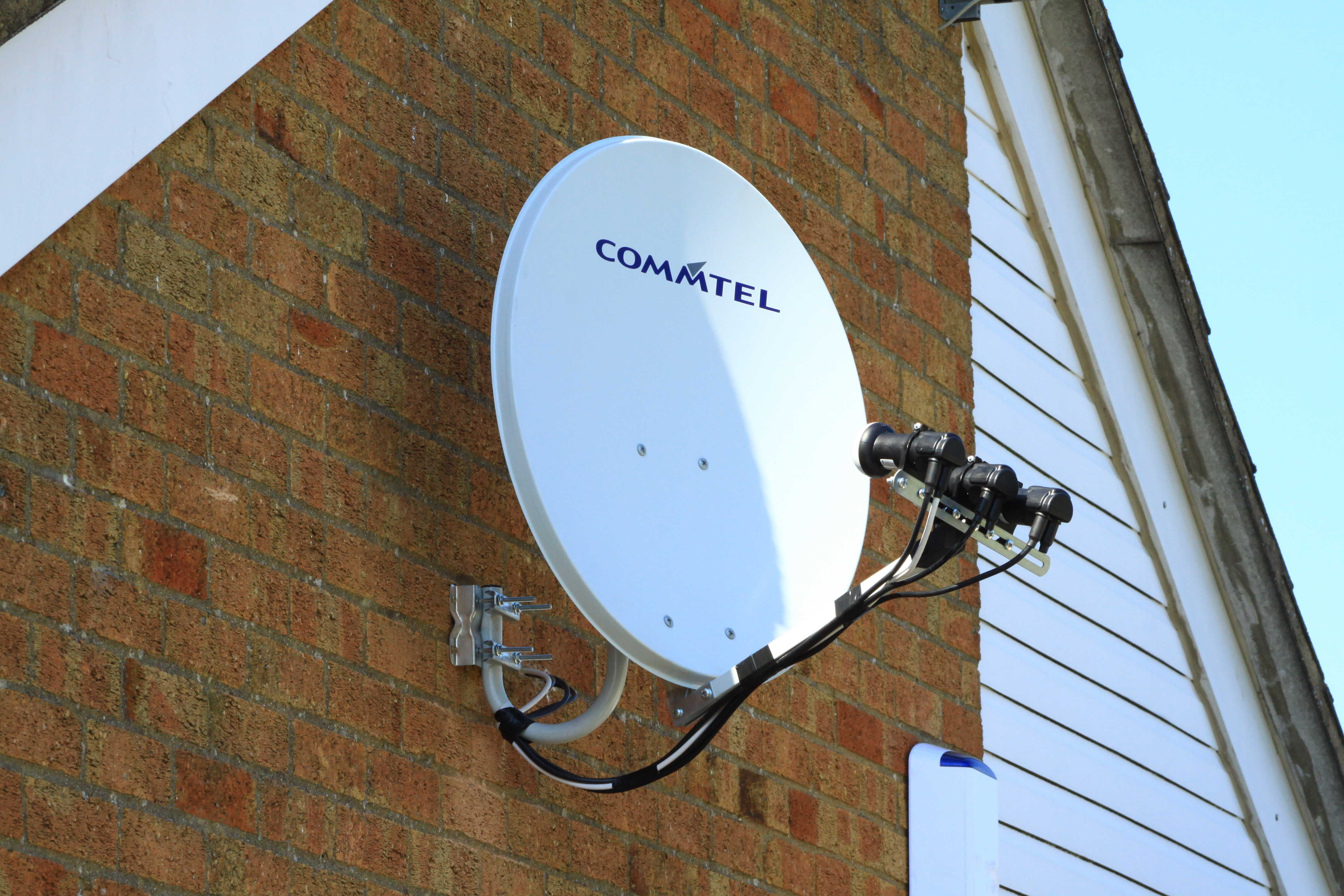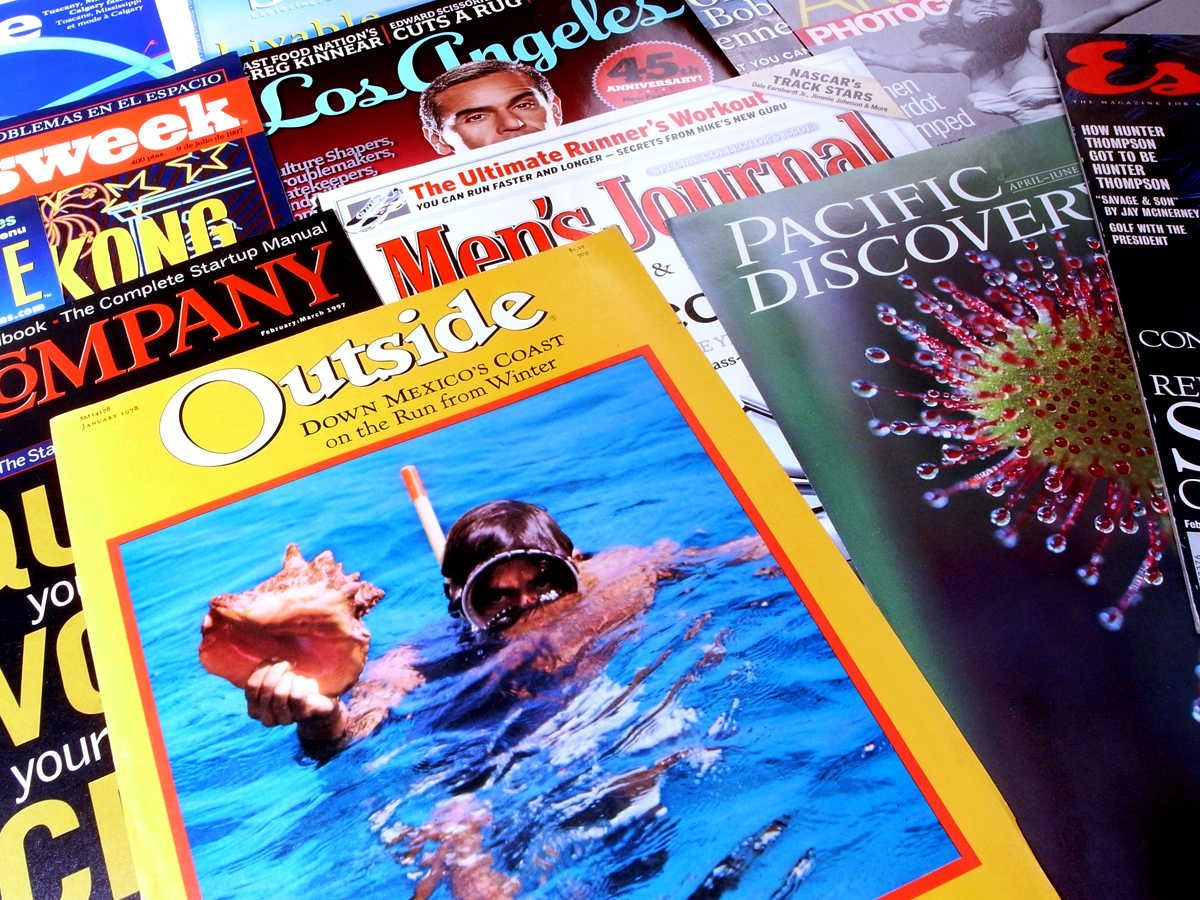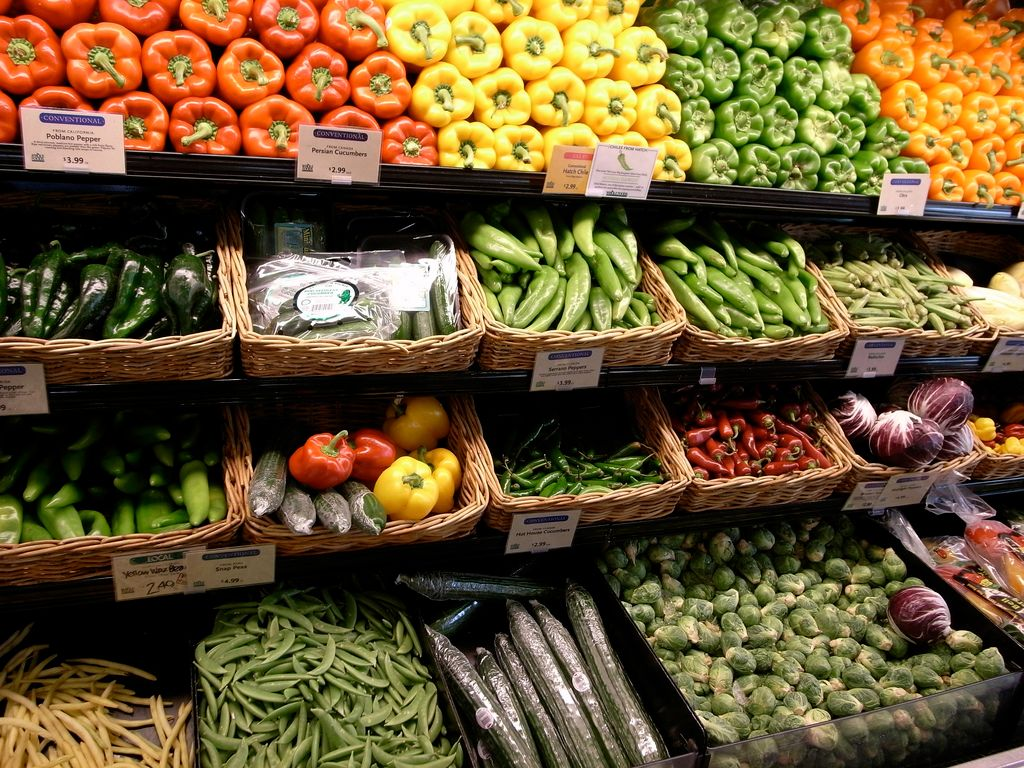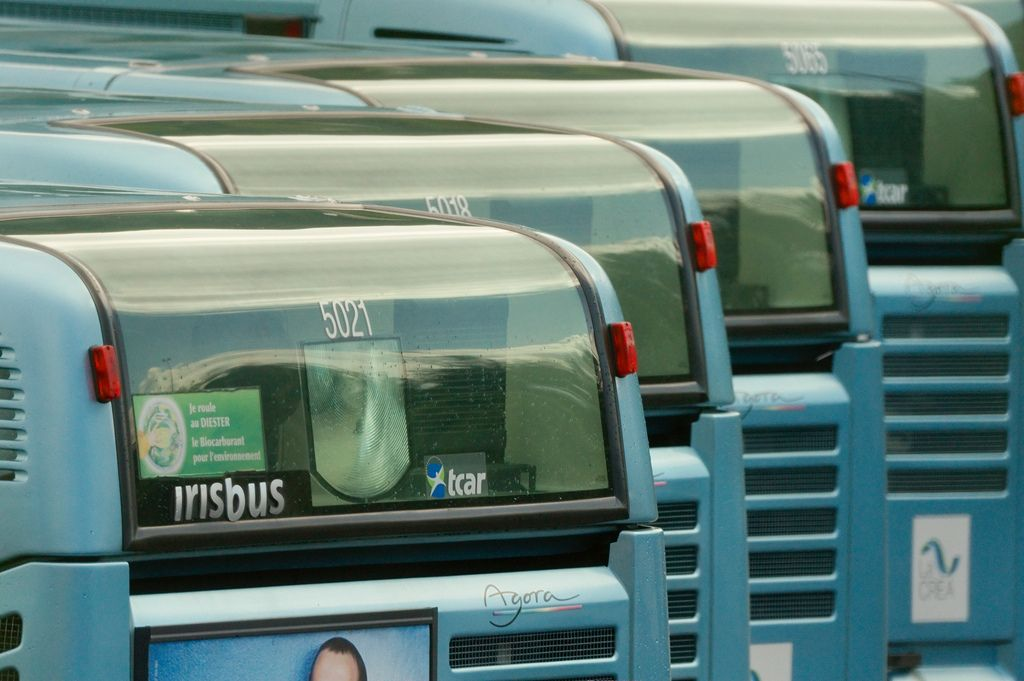Home Practice
For learners and parents For teachers and schools
Textbooks
Full catalogue
Pricing SupportLog in

We think you are located in United States. Is this correct?

# 8.3 Personal budgets, and income and expenditure statements

## 8.3 Personal budgets, and income and expenditure statements (EMG5G)

Drawing up a budget is a good way to plan your expenditure based on how much money you expect to receive. Budgets are often estimates, because you cannot easily include unexpected expenses in a budget. However, you can plan to save some money that you can use in the event of an unexpected expense. Budgeting includes normal monthly budgeting and budgeting for particular expenses, such as an expensive item that you want to buy, a project or a trip overseas.

### Monthly budgeting (EMG5H)

To produce a budget you need to estimate what your income and expenses should be for a given month. You might already know exactly how much your income is, if it is fixed.

Your budget should help you to get the most out of your money and will help to enable you to have enough money to cover all your expenses.

There are several things you should aim for in your personal budget:

• It should list all of the items that are needed and should try to anticipate unforeseen expenses.
• It should be realistic, so that you can keep to it.
• It should focus on the high priority items (essential items such as food and health care). If too much of the income is spent on non-essential items and not on savings, your budget is going to become problematic in the future.
• An ideal budget should include a plan to save money for the future, or to pay off debts to allow for savings in the following months.
• It should be balanced. If your income is less than your expenses, then you need to revise it until the two sides balance. If your income is more than your expenses, then you should plan to save the extra money.

## Managing a personal monthly budget

Exercise 8.3

Jacob is a young man in his first job. He earns a salary of $$\text{R}\,\text{5 900}$$. He has the following expenses:

 Rent $$\text{R}\,\text{1 500}$$ Clothing $$\text{R}\,\text{260}$$ Water and lights $$\text{R}\,\text{280}$$ Satellite TV subscription$$\text{R}\,\text{280}$$ Taxi transport $$\text{R}\,\text{900}$$ Groceries $$\text{R}\,\text{940}$$ Cell phone contract $$\text{R}\,\text{99}$$ Magazines$$\text{R}\,\text{180}$$ Instalment on DVD player $$\text{R}\,\text{350}$$ Bank charges $$\text{R}\,\text{52}$$ Entertainment $$\text{R}\,\text{580}$$ Medicine$$\text{R}\,\text{120}$$

Classify each item of his expenses as high priority or low priority.

High priority: Rent, water and lights, groceries, taxi transport, bank charges, medicine, cell phone contract, instalment on DVD player. Low priority: Clothing, satellite TV subscription, magazines, entertainment.

Calculate the total cost of his variable expenses.

Variable expenses include clothing, water and lights, taxi transport, groceries, magazines, entertainment. So: $$\text{R}\,\text{260}$$ + $$\text{R}\,\text{280}$$ + $$\text{R}\,\text{900}$$ + $$\text{R}\,\text{940}$$ + $$\text{R}\,\text{180}$$ + $$\text{R}\,\text{340}$$ + $$\text{R}\,\text{580}$$ = $$\text{R}\,\text{3 480}$$

Jacob has no savings plan and lives just within his income.

Write down a few things that could happen that would make him unable to live within his income.

His rent could increase. The cost of his medicine may increase. The cost of taxi transport could increase.

What expenses could he reduce to help him to save up for unforeseen expenses?

He could reduce his spending on clothing, entertainment and magazines, and possibly cancel his satellite subscription.

Suppose now that Jacob earns $$\text{R}\,\text{6 500}$$ each month. He could choose to buy a flat screen TV on hire purchase but he still owes $$\text{R}\,\text{2 000}$$ on the DVD player. What would you advise him to do?Jacob should finish paying for the DVD player before he acquires more debt.

### Budgeting for particular items or events (EMG5J)

In this section we look at situations in which we need to plan for a certain event, rather than for monthly expenses.

## Worked example 3: Budgeting for a party

1. Jennifer is planning to have a party. She is going to charge a small fee ($$\text{R}\,\text{20}$$ per person) at the door to help cover her costs. She expects $$\text{50}$$ friends to come to the party. She is also going to spend some of her own money on the catering. Jennifer draws up a budget and anticipates that her income and expenditure will be as follows:Income Total Cover charge at door $$\text{R}\,\text{20}$$ $$\times$$ $$\text{50}$$ friends $$\text{R}\,\text{1 000,00}$$ Jennifer's contribution $$\text{R}\,\text{400}$$ $$\text{R}\,\text{1 400,00}$$
 Expenditure Total Juice $$\text{R}\,\text{200}$$ $$\text{R}\,\text{200,00}$$ Cake $$\text{R}\,\text{300}$$ $$\text{R}\,\text{500,00}$$ Paper plates and cups $$\text{R}\,\text{100}$$ $$\text{R}\,\text{600,00}$$ $$\text{R}\,\text{600,00}$$
1. Can you think of any other expenses she has forgotten to include in her budget?
2. If all $$\text{50}$$ friends arrive and Jennifer sticks to her budget of $$\text{R}\,\text{600}$$ worth of expenses, how much money will she have left over?
3. At the last minute, Jennifer decides to hire a DJ to play music at her party. The DJ charges her $$\text{R}\,\text{400}$$. Does she have enough money to cover this expense? And how much money will be left once she's paid the DJ?
4. SITUATION A: Jennifer is expecting $$\text{50}$$ friends to come to the party but only $$\text{9}$$ arrive. How much money does she now have and can she still afford her expenses?

5. SITUATION B: Jennifer expected $$\text{50}$$ friends and $$\text{48}$$ friends arrived.

1. How much money will she make from the cover charge?
2. Copy the income and expenditure tables given above and fill in the new information (including the money for the DJ). Calculate the new totals.
3. What do you think Jennifer should do with the money left over? Discuss with your class.
2. It is Thabo's birthday next month and he wants to have a party. He needs to think about how much it is going to cost. His mom suggests that he send out party invitations. The invitations would include details of the date, time, party venue and contact details, with a request to reply (RSVP) date. Printing the invitations would cost $$\text{R}\,\text{80}$$. Based on what you learnt about budgets, would you advise Thabo to send invitations? Discuss with the rest of your class.1. Examples could include streamers and balloons, hiring a sound system, etc.
2. $$\text{R}\,\text{1 400} - \text{R}\,\text{600} = \text{R}\,\text{800}$$.
3. Expenses = $$\text{R}\,\text{600}$$ + $$\text{R}\,\text{400}$$ = $$\text{R}\,\text{1 000}$$. She will have $$\text{R}\,\text{400}$$ left once she's paid the DJ.
4. $$\text{R}\,\text{9} \times \text{20} = \text{R}\,\text{180}$$. $$\text{R}\,\text{180}$$ from friends + $$\text{R}\,\text{400}$$ from Jennifer's savings = $$\text{R}\,\text{580}$$. Therefore Jennifer will not have enough money to cover her expenses. She will need to pay $$\text{R}\,\text{20}$$ more.
1. $$\text{R}\,\text{20}\times \text{48} = \text{R}\,\text{960}$$
2.  Income Total Cover change at door $$\text{R}\,\text{20}$$ $$\times$$ $$\text{50}$$ friends $$\text{R}\,\text{960,00}$$ Jennifer's contribution $$\text{R}\,\text{400}$$ $$\text{R}\,\text{1 360,00}$$
 Expenditure Running total Juice $$\text{R}\,\text{200}$$ $$\text{R}\,\text{200,00}$$ Cake $$\text{R}\,\text{300}$$ $$\text{R}\,\text{500,00}$$ Paper plates and cups $$\text{R}\,\text{100}$$ $$\text{R}\,\text{600,00}$$ DJ $$\text{R}\,\text{400}$$ $$\text{R}\,\text{1 000}$$ $$\text{R}\,\text{1 000,00}$$
3. Open for discussion. She could keep the money so that she does not need to put forward $$\text{R}\,\text{400}$$. She could take the total money left over and divide it between all the friends who came to the party.
1. If Thabo gave out invitations, he would have a better idea of how many people were coming then he will be able to make a more accurate estimate of how much food to buy and how much money he can spend.He will therefore be less likely to overspend.

## Understanding a budget

Exercise 8.4

Study the budget below and answer the questions that follow: Sam is currently in Grade 12 and he works part-time at Checkers Hypermarket to earn money. Sam's monthly budget is as follows:

 Budget Expenditure Income Earnings from Checkers $$\text{R}\,\text{800}$$ Allowance from parents $$\text{R}\,\text{200}$$ Transport $$\text{R}\,\text{80}$$ Food$$\text{R}\,\text{160}$$ Entertainment $$\text{R}\,\text{200}$$ Clothes $$\text{R}\,\text{180}$$ Unforeseen expenses $$\text{R}\,\text{100}$$ Interest received from fixed account deposits $$\text{R}\,\text{50}$$ Investment in fixed account $$\text{R}\,\text{100}$$ Present for girlfriend$$\text{R}\,\text{100}$$ Total $$\text{R}\,\text{920}$$ $$\text{R}\,\text{1 050}$$ Surplus or deficit Income - Expenditure $$\text{R}\,\text{1 050}$$ - $$\text{920}$$ = $$\text{130}$$

Estimate what fraction of total expenditure he plans to spend on clothes.

The fraction of total expenditure to be spent on clothes =$$\frac{\text{180}}{\text{920}} = \frac{\text{9}}{\text{46}}$$

$$\frac{\text{9}}{\text{46}} \times \text{100} = \text{19,56} \%$$

Estimate as a fraction of total income the amount of money he earns at Checkers. Express it as a percentage of total income.$$\frac{\text{800}}{\text{1 050}} = \frac{\text{16}}{\text{21}}$$, and as a percentage of the total income $$= \frac{\text{16}}{\text{21}} \times \text{100} = \text{76,19} \%$$

Thando and Lisa are thinking of going on a planned adventure hike. They find the following advertisement from a company who arrange hikes.Draw up a budget for both of them, assuming that they do not have any equipment of their own.

Douglas wants to travel from Cape Town to Durban to visit his cousin. His parents said that they can give him $$\text{R}\,\text{500}$$ towards the trip. He decides to draw up a budget to determine how much money the trip will cost. His uncle has offered to give him a lift home so he only needs to budget for the trip to Durban. He has $$\text{R}\,\text{2 000}$$ saved in his bank account. He wants to have some spending money left over when he gets there.

He phones Rainbow Buses to find out how much it costs to travel from Cape Town to Durban. They give him two options:

OPTION 1: Leave Saturday morning and travel straight to Durban. The trip costs $$\text{R}\,\text{1 200}$$ and he will need to pay for $$\text{3}$$ meals at $$\text{R}\,\text{30}$$ per meal

OPTION 2: Leave Saturday morning and travel to Plettenberg Bay first. The trip costs only $$\text{R}\,\text{400}$$. He can then catch a bus on Sunday morning to Durban. This bus trip will cost $$\text{R}\,\text{500}$$. If he does this he needs to find a place to stay on Saturday night and budget for three extra meals (estimated at $$\text{R}\,\text{30}$$ each). He estimates that a Backpackers' Lodge would be the cheapest place to stay, at $$\text{R}\,\text{200}$$ a night.

 Income Expenses Running total of money that he has Money from parents Savings Bus fare Meals on bus Accommodation

Copy the above budget sheet and fill in the amounts for income and expenses in the correct columns for:

1. Option 1.
2. Option 2.

OPTION 1:

 Income Expenses Running total Money from parents $$\text{500}$$ $$\text{500}$$ Savings $$\text{2 000}$$ $$\text{2 500}$$ Bus fare $$\text{1 200}$$ $$\text{1 300}$$ Meals on bus $$\text{3}\times \text{30} = \text{90}$$ $$\text{1 210}$$ Accommodation $$\text{0}$$ $$\text{1 210}$$

OPTION 2:

 Income Expenses Running total Money from parents $$\text{500}$$ $$\text{500}$$ Savings $$\text{2 000}$$ $$\text{2 500}$$ Bus fare $$\text{400}$$ + $$\text{500}$$ = $$\text{900}$$ $$\text{1 600}$$ Meals on bus $$\text{6} \times \text{30} = \text{180}$$ $$\text{1 420}$$ Accommodation $$\text{200}$$ $$\text{1 220}$$